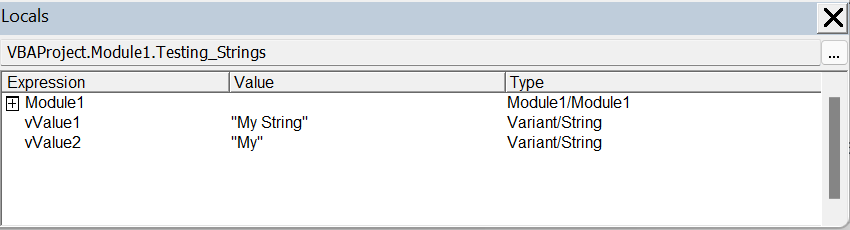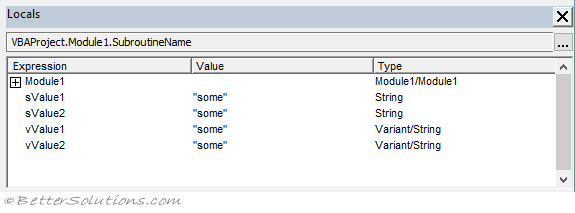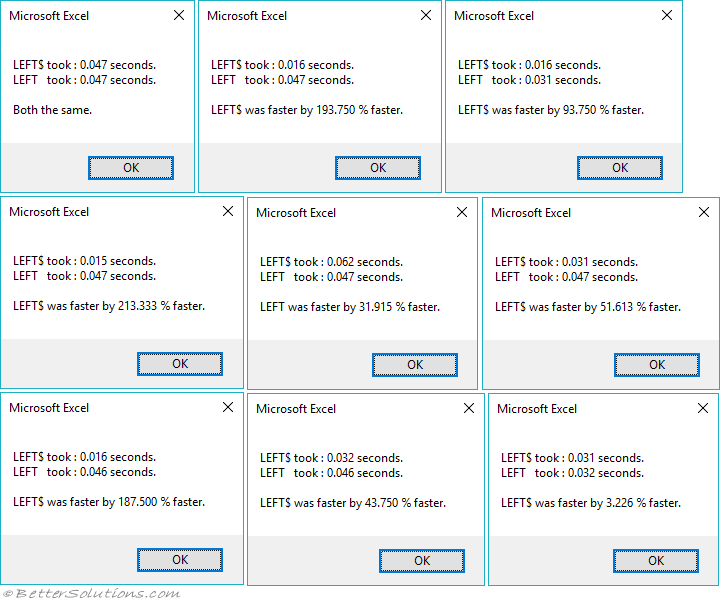### String-Variant

The variant has a special sub type called Variant/String that can be used to hold variable-length strings.

`Dim vValue1 As Variant Dim vValue2 As Variant vValue1 = "My String" vValue2 = Left\$("My String", 2) `#### Variant/String

As well as fixed-length strings and variable-length strings there is also a variant string subtype.
Also called a Variant-Character data type.
This data type as its name suggests is a sub type of the variant data type.
This data type uses 22 bytes plus the length of the string.
This data type is much larger than a normal variable-length string.
The string-variant data type can contain the same as a variable-length string.
The default value is Empty.

#### String Functions

All String Functions can accept String variables and Variant/String variables.
Some string functions return String data types and some return Variant/String data types.
LEFT - Returns a number of characters from the left of a string (Variant/String).
LEFT\$ - Returns a number of characters from the left of a string (String).
If you declare your variable as a String you get a String data type from both functions.
If you declare your variable as a Variant you get a Variant/String data type from both functions.

#### Dollar Functions - String Data Type

The string functions that can return both String and Variant/String are listed on the Dollar Functions page.
If you declare your variable as a String and use the LEFT function, a conversion from Variant/String to String is performed (automatically).
For most of us this is absolutely fine and something we would not even be aware of.

`Dim sValue1 As String sValue1 = Left("some text", 4) `

For some of us though, this unnecessary data type conversion (from Variant/String to String) can be removed.
If you declare your variable as a String and use the LEFT\$ function, the conversion does not take place.

`Dim sValue2 As String sValue2 = Left\$("some text", 4) `

#### Dollar Functions - Variant Data Type

If you declare your variable as a Variant and use the LEFT function, a conversion does not take place.
If you declare your variable as a Variant and use the LEFT\$ function, a conversion from String to Variant/String is performed (automatically).

`Dim vValue1 As Variant Dim vValue2 As Variant vValue1 = Left("some text", 4) vValue2 = Left\$("some text", 4) `

#### Locals Window

It is possible to see these different data types by using the Locals Window.You cannot use the TYPENAME function to see the different data types.
Whether you are declaring your variables as Strings or Variants the string returned from TYPENAME is "String" in both cases.

`Dim sValue1 As String Dim vValue1 As Variant Debug.Print TypeName(sValue1)   'String  Debug.Print TypeName(vValue1)   'String  `

#### Which Is Faster ?

There are rumours that the Dollar Functions are faster but this is really not worth worrying about.
There is definitely a difference in speed when you are iterating through a very large number of times.
Unless you are iterating through hundreds of thousands of times, there is no difference.

`Declare PtrSafe Function GetTickCount Lib "kernel32.dll" () As Long Sub TimingSubroutine() Dim iloop As Integer Dim lRow As Long Dim dbTime As Double Dim sShort As String Dim left_nodollar As Single Dim left_dollar As Single Dim percentage As Single         For iloop = 1 To 10         dbTime = GetTickCount()         For lRow = 1 To 300000   '300,000 times              sShort = Left\$("some text", 4)         Next lRow         left_dollar = (GetTickCount() - dbTime) / 1000                 dbTime = GetTickCount()         For lRow = 1 To 300000   '300,000 times              sShort = Left("some text", 4)         Next lRow         left_nodollar = (GetTickCount() - dbTime) / 1000             If ((left_dollar > 0) And (left_nodollar > 0)) Then             If (left_nodollar > left_dollar) Then                 percentage = ((left_nodollar - left_dollar) / left_dollar) * 100                             Call MsgBox("LEFT\$ took : " & left_dollar & " seconds." & vbCrLf & _                             "LEFT   took : " & left_nodollar & " seconds." & vbCrLf & vbCrLf & _                             "LEFT\$ was faster by " & VBA.Format(percentage, "0.000") & " % faster.")             ElseIf (left_dollar > left_nodollar) Then                 percentage = ((left_dollar - left_nodollar) / left_nodollar) * 100                             Call MsgBox("LEFT\$ took : " & left_dollar & " seconds." & vbCrLf & _                             "LEFT   took : " & left_nodollar & " seconds." & vbCrLf & vbCrLf & _                             "LEFT was faster by " & VBA.Format(percentage, "0.000") & " % faster.")             Else                 Call MsgBox("LEFT\$ took : " & left_dollar & " seconds." & vbCrLf & _                             "LEFT   took : " & left_nodollar & " seconds." & vbCrLf & vbCrLf & _                             "Both the same.")             End If         Else             Call MsgBox("One of the times is zero.")         End If     Next iloop End Sub `